# 万字教你如何用 Python 实现线性规划

• 2021 年 12 月 17 日
• 本文字数：12948 字

阅读完需：约 42 分钟

## 线性规划说明

### 为什么线性规划很重要？

• Gurobi 优化案例研究

• 线性规划技术的五个应用领域

### 使用 Python 进行线性规划

Python 适合围绕本机库构建包装器，因为它可以很好地与 C/C++ 配合使用。对于本教程，您不需要任何 C/C++（或 Fortran），但如果您想了解有关此酷功能的更多信息，请查看以下资源：

• 构建 Python C 扩展模块

• CPython 内部

• 用 C 或 C++ 扩展 Python

• SciPy Optimization and Root Finding

• PuLP

• Pyomo

• CVXOPT

## 线性规划示例

1. 一个说明什么是线性规划的小问题

2. 一个与资源分配相关的实际问题，它说明了现实世界场景中的线性规划概念

### 小型线性规划问题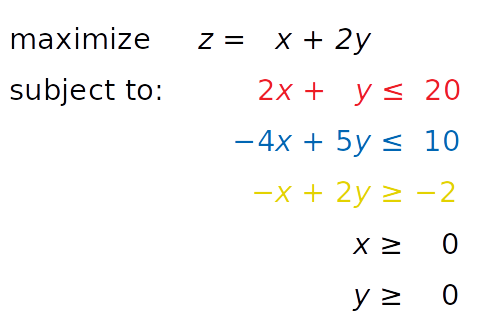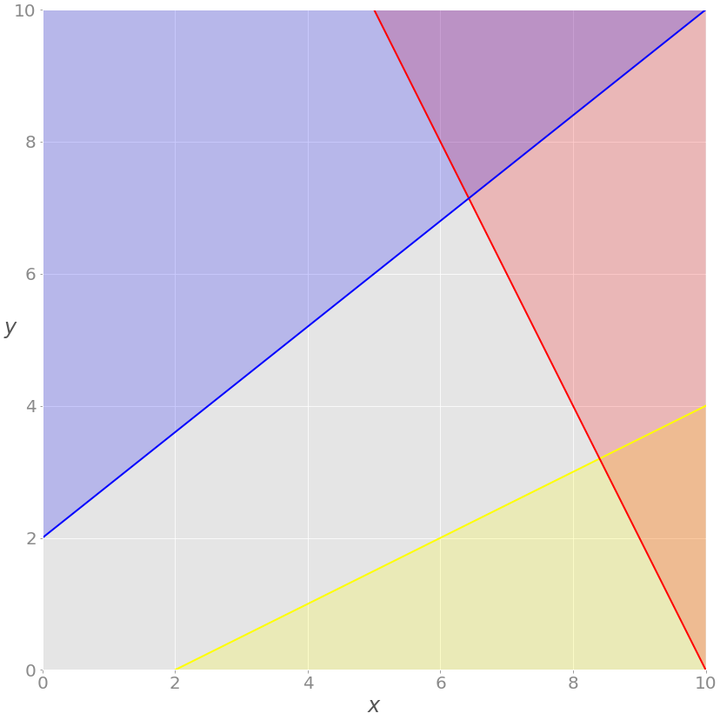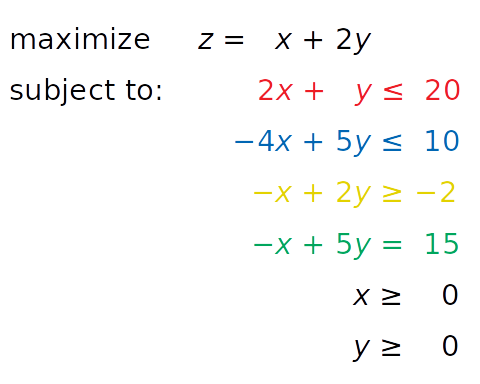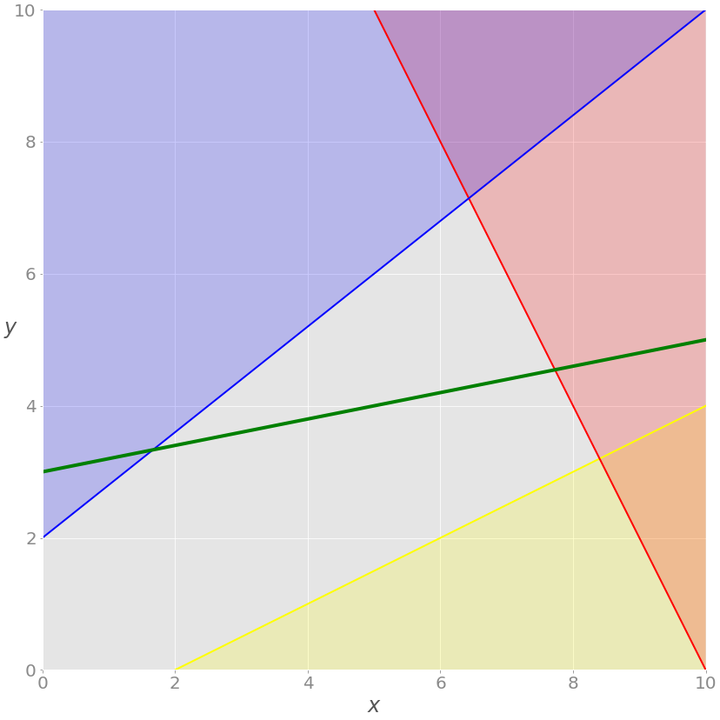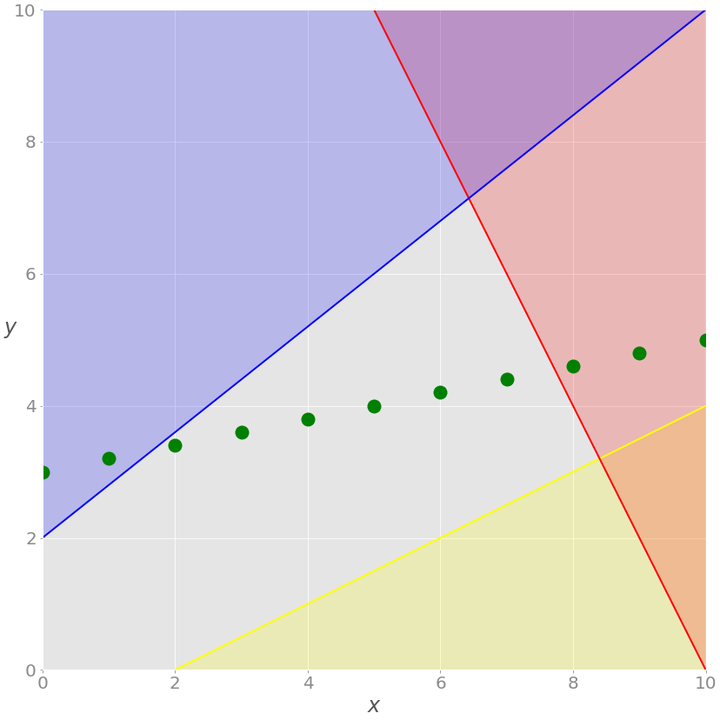### 资源分配问题

1. 第一种、第二种、第三种和第四种产品的每单位产品利润分别为 20 美元、12 美元、40 美元和 25 美元。

2. 由于人力限制，每天生产的总数量不能超过五十台。

3. 对于每单位第一个产品，消耗三个单位的原材料 A。每单位第二产品需要两单位原料 A 和一单位原料 B。每单位第三产品需要一单位 A 和两单位 B。最后，每单位第四产品需要三 B 的单位

4. 由于运输和储存的限制，工厂每天最多可以消耗一百单位的原材料 A 和九十单位的 B。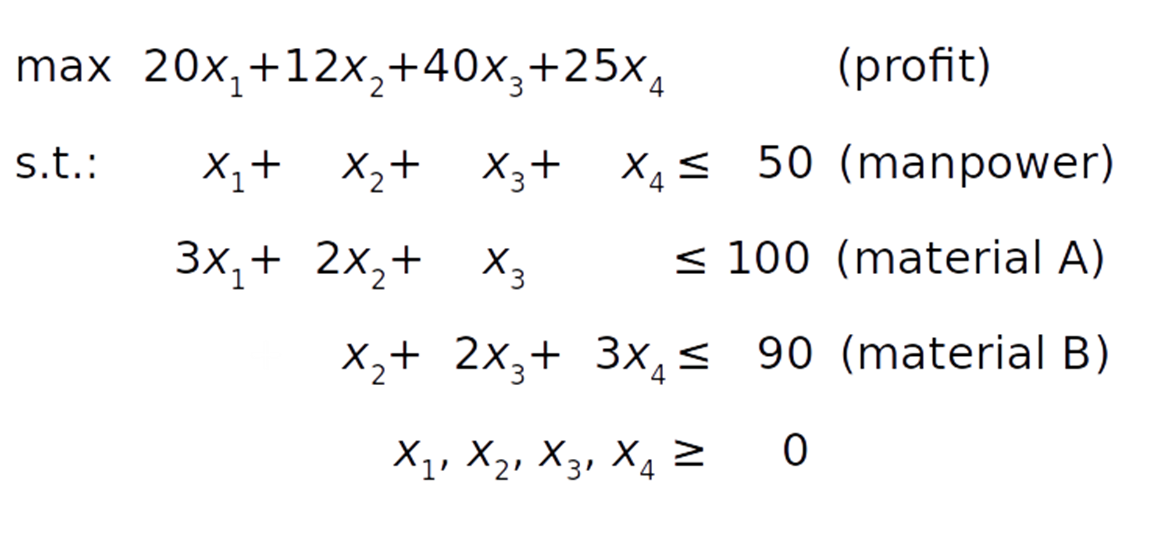## 线性规划 Python 实现

1. SciPy 是一个用于使用 Python 进行科学计算的通用包。

2. PuLP 是一个 Python 线性编程 API，用于定义问题和调用外部求解器。

SciPy 设置起来很简单。安装后，您将拥有开始所需的一切。它的子包 scipy.optimize 可用于线性和非线性优化。

PuLP 允许您选择求解器并以更自然的方式表述问题。PuLP 使用的默认求解器是 COIN-OR Branch and Cut Solver (CBC)。它连接到用于线性松弛的 COIN-OR 线性规划求解器 (CLP)和用于切割生成的 COIN-OR 切割生成器库 (CGL)。

### 安装 SciPy 和 PuLP

$python -m pip install -U "scipy==1.4.*" "pulp==2.1" 复制代码 您可能需要运行 pulptest 或 sudo pulptest 启用 PuLP 的默认求解器，尤其是在您使用 Linux 或 Mac 时： $ pulptest

$brew install glpk 复制代码 在 Debian 和 Ubuntu 上，使用 apt 来安装 glpk 和 glpk-utils： $ sudo apt install glpk glpk-utils

$sudo dnf install glpk-utils 复制代码 您可能还会发现 conda 对安装 GLPK 很有用： $ conda install -c conda-forge glpk

\$ glpsol --version

### 使用 SciPy

>>>>>> from scipy.optimize import linprog

### 示例 1linprog()仅解决最小化（而非最大化）问题，并且不允许具有大于或等于符号 (≥) 的不等式约束。要解决这些问题，您需要在开始优化之前修改您的问题：

• 不是最大化 z = x + 2 y，你可以最小化它的负值（− z = − x − 2 y）。

• 代替大于或等于符号，您可以将黄色不等式乘以 -1 并得到小于或等于符号 (≤) 的相反数。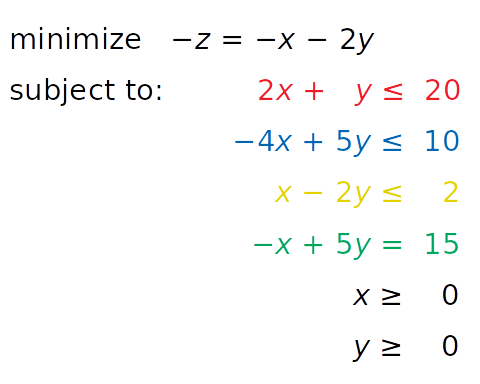>>>>>> obj = [-1, -2]>>> #      ─┬  ─┬>>> #       │   └┤ Coefficient for y>>> #       └────┤ Coefficient for x>>> lhs_ineq = [[ 2,  1],  # Red constraint left side...             [-4,  5],  # Blue constraint left side...             [ 1, -2]]  # Yellow constraint left side>>> rhs_ineq = [20,  # Red constraint right side...             10,  # Blue constraint right side...              2]  # Yellow constraint right side>>> lhs_eq = [[-1, 5]]  # Green constraint left side>>> rhs_eq =        # Green constraint right side

• obj 保存目标函数的系数。

• lhs_ineq 保存不等式（红色、蓝色和黄色）约束的左侧系数。

• rhs_ineq 保存不等式（红色、蓝色和黄色）约束的右侧系数。

• lhs_eq 保存来自等式（绿色）约束的左侧系数。

• rhs_eq 保存来自等式（绿色）约束的右侧系数。

>>>>>> bnd = [(0, float("inf")),  # Bounds of x...        (0, float("inf"))]  # Bounds of y

>>>>>> opt = linprog(c=obj, A_ub=lhs_ineq, b_ub=rhs_ineq,...               A_eq=lhs_eq, b_eq=rhs_eq, bounds=bnd,...               method="revised simplex")>>> opt     con: array([0.])     fun: -16.818181818181817 message: 'Optimization terminated successfully.'     nit: 3   slack: array([ 0.        , 18.18181818,  3.36363636])  status: 0 success: True       x: array([7.72727273, 4.54545455])

1. method="interior-point"选择内点法。默认情况下设置此选项。

2. method="revised simplex" 选择修正的两相单纯形法。

3. method="simplex" 选择传统的两相单纯形方法。

linprog() 返回具有以下属性的数据结构：

• .con 是等式约束残差。

• .fun 是最优的目标函数值（如果找到）。

• .message 是解决方案的状态。

• .nit 是完成计算所需的迭代次数。

• .slack 是松弛变量的值，或约束左右两侧的值之间的差异。

• .status 是一个介于 0 和之间的整数 4，表示解决方案的状态，例如 0 找到最佳解决方案的时间。

• .success 是一个布尔值，显示是否已找到最佳解决方案。

• .x 是一个保存决策变量最优值的 NumPy 数组。

>>>>>> opt.fun-16.818181818181817>>> opt.successTrue>>> opt.xarray([7.72727273, 4.54545455])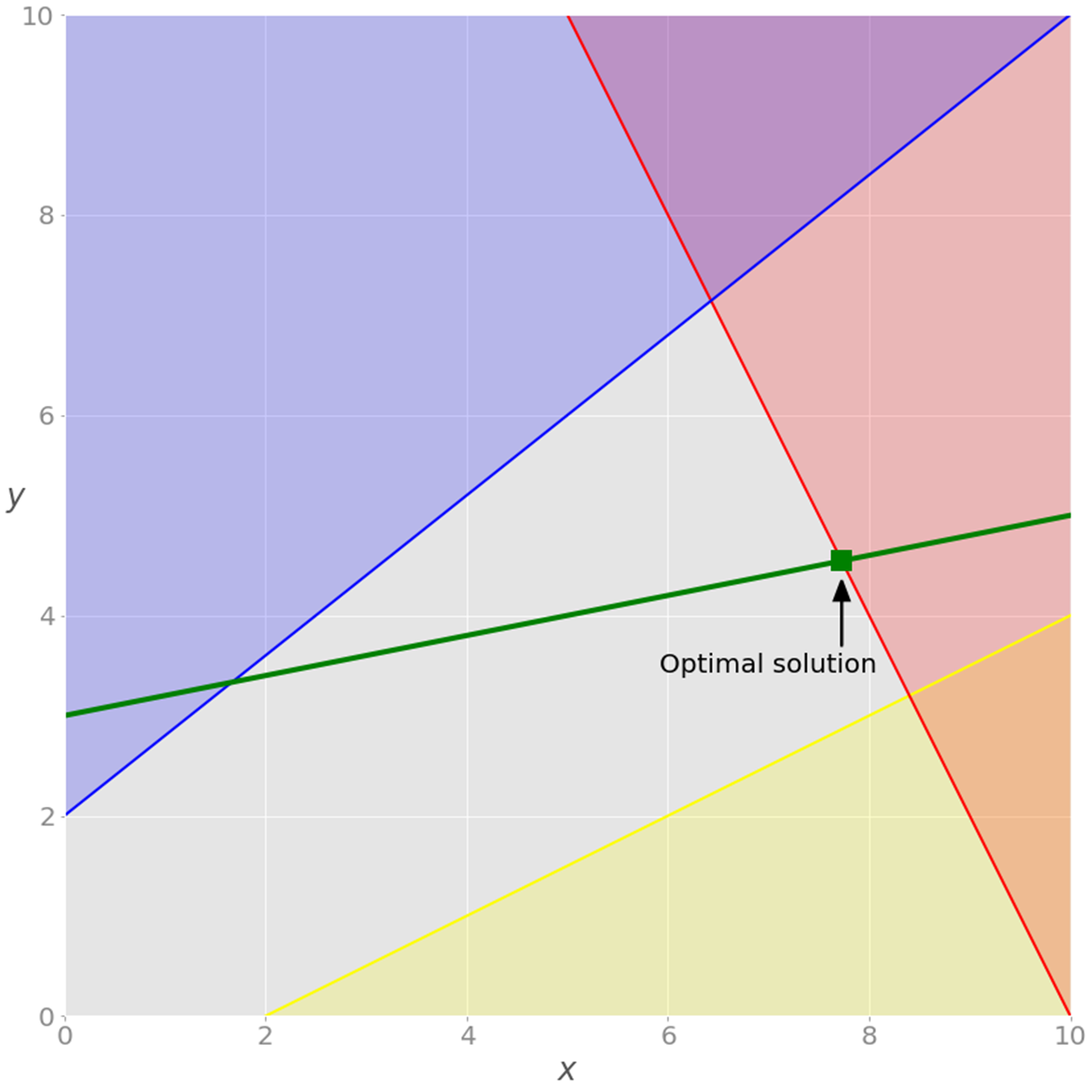>>>>>> opt = linprog(c=obj, A_ub=lhs_ineq, b_ub=rhs_ineq, bounds=bnd,...               method="revised simplex")>>> opt     con: array([], dtype=float64)     fun: -20.714285714285715 message: 'Optimization terminated successfully.'     nit: 2   slack: array([0.        , 0.        , 9.85714286])  status: 0 success: True       x: array([6.42857143, 7.14285714]))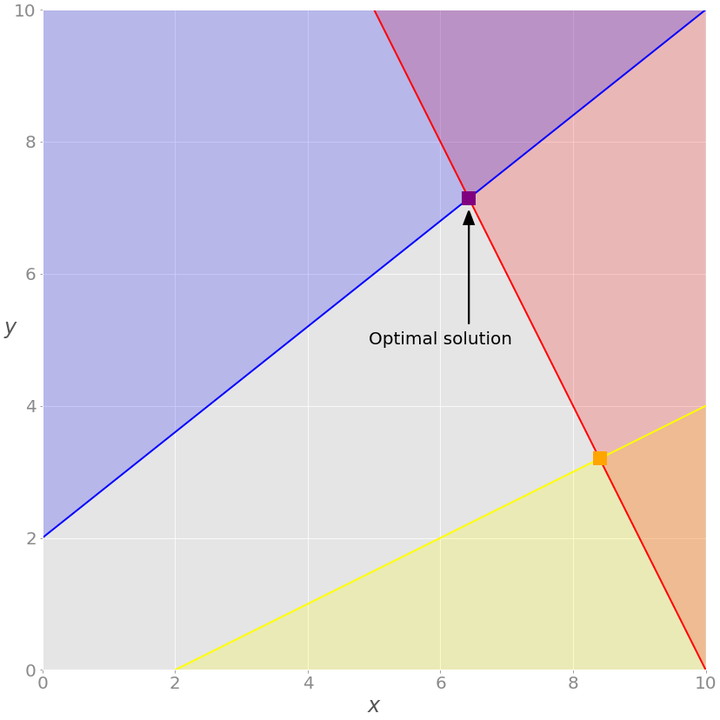### 示例 2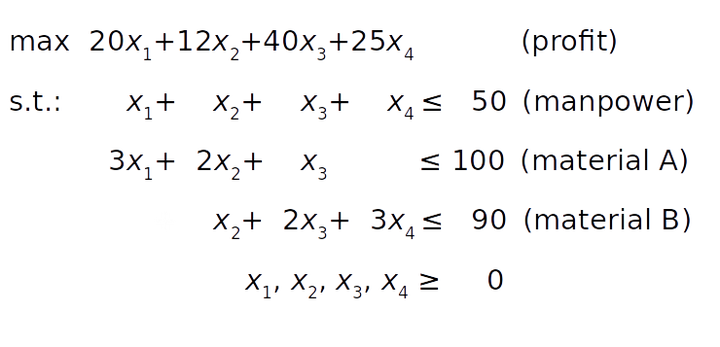>>>>>> obj = [-20, -12, -40, -25]>>> lhs_ineq = [[1, 1, 1, 1],  # Manpower...             [3, 2, 1, 0],  # Material A...             [0, 1, 2, 3]]  # Material B>>> rhs_ineq = [ 50,  # Manpower...             100,  # Material A...              90]  # Material B>>> opt = linprog(c=obj, A_ub=lhs_ineq, b_ub=rhs_ineq,...               method="revised simplex")>>> opt     con: array([], dtype=float64)     fun: -1900.0 message: 'Optimization terminated successfully.'     nit: 2   slack: array([ 0., 40.,  0.])  status: 0 success: True       x: array([ 5.,  0., 45.,  0.])

1. 第三个产品带来的单位利润最大，因此工厂将生产最多。

2. 第一个 slack 是 0，表示人力（第一）约束左右两边的值是一样的。工厂每天 50 生产单位，这是它的全部产能。

3. 第二个松弛是 40 因为工厂消耗了 60 单位的原材料 A（第一种产品为 15 单位，第三种产品为 45100 单位）。

4. 第三个裕量是 0，这意味着工厂消耗了所有 90 单位的原材料 B。这全部量都用于第三个产品。这就是为什么工厂根本不能生产第二或第四种产品，也不能生产超过 45 单位的第三种产品。缺乏原材料 B.

opt.statusis0 和 opt.successis True，说明优化问题成功求解，最优可行解。

SciPy 的线性规划功能主要用于较小的问题。对于更大和更复杂的问题，您可能会发现其他库更适合，原因如下：

• SciPy 无法运行各种外部求解器。

• SciPy 不能使用整数决策变量。

• SciPy 不提供促进模型构建的类或函数。您必须定义数组和矩阵，这对于大型问题来说可能是一项乏味且容易出错的任务。

• SciPy 不允许您直接定义最大化问题。您必须将它们转换为最小化问题。

• SciPy 不允许您直接使用大于或等于符号来定义约束。您必须改用小于或等于。

### Using PuLP

PuLP 具有比 SciPy 更方便的线性编程 API。您不必在数学上修改您的问题或使用向量和矩阵。一切都更干净，更不容易出错。

from pulp import LpMaximize, LpProblem, LpStatus, lpSum, LpVariable

### 示例 1# Create the modelmodel = LpProblem(name="small-problem", sense=LpMaximize)

# Initialize the decision variablesx = LpVariable(name="x", lowBound=0)y = LpVariable(name="y", lowBound=0)

>>>>>> expression = 2 * x + 4 * y>>> type(expression)<class 'pulp.pulp.LpAffineExpression'>>>> constraint = 2 * x + 4 * y >= 8>>> type(constraint)<class 'pulp.pulp.LpConstraint'>

# Add the constraints to the modelmodel += (2 * x + y <= 20, "red_constraint")model += (4 * x - 5 * y >= -10, "blue_constraint")model += (-x + 2 * y >= -2, "yellow_constraint")model += (-x + 5 * y == 15, "green_constraint")

# Add the objective function to the modelobj_func = x + 2 * ymodel += obj_func

# Add the objective function to the modelmodel += x + 2 * y

# Add the objective function to the modelmodel += lpSum([x, 2 * y])

>>>>>> modelsmall-problem:MAXIMIZE1*x + 2*y + 0SUBJECT TOred_constraint: 2 x + y <= 20blue_constraint: 4 x - 5 y >= -10yellow_constraint: - x + 2 y >= -2green_constraint: - x + 5 y = 15VARIABLESx Continuousy Continuous

# Solve the problemstatus = model.solve()

.solve()调用底层求解器，修改 model 对象，并返回解决方案的整数状态，1 如果找到了最优解。有关其余状态代码，请参阅 LpStatus[]。

>>>>>> print(f"status: {model.status}, {LpStatus[model.status]}")status: 1, Optimal>>> print(f"objective: {model.objective.value()}")objective: 16.8181817>>> for var in model.variables():...     print(f"{var.name}: {var.value()}")...x: 7.7272727y: 4.5454545>>> for name, constraint in model.constraints.items():...     print(f"{name}: {constraint.value()}")...red_constraint: -9.99999993922529e-08blue_constraint: 18.181818300000003yellow_constraint: 3.3636362999999996green_constraint: -2.0000000233721948e-07)

model.objective 持有目标函数 model.constraints 的值，包含松弛变量的值，以及对象 x 和 y 具有决策变量的最优值。model.variables()返回一个包含决策变量的列表：

>>>>>> model.variables()[x, y]>>> model.variables() is xTrue>>> model.variables() is yTrue

>>>>>> model.solver<pulp.apis.coin_api.PULP_CBC_CMD object at 0x7f60aea19e50>

from pulp import GLPK

# Create the modelmodel = LpProblem(name="small-problem", sense=LpMaximize)# Initialize the decision variablesx = LpVariable(name="x", lowBound=0)y = LpVariable(name="y", lowBound=0)# Add the constraints to the modelmodel += (2 * x + y <= 20, "red_constraint")model += (4 * x - 5 * y >= -10, "blue_constraint")model += (-x + 2 * y >= -2, "yellow_constraint")model += (-x + 5 * y == 15, "green_constraint")# Add the objective function to the modelmodel += lpSum([x, 2 * y])# Solve the problemstatus = model.solve(solver=GLPK(msg=False))

>>>>>> print(f"status: {model.status}, {LpStatus[model.status]}")status: 1, Optimal>>> print(f"objective: {model.objective.value()}")objective: 16.81817>>> for var in model.variables():...     print(f"{var.name}: {var.value()}")...x: 7.72727y: 4.54545>>> for name, constraint in model.constraints.items():...     print(f"{name}: {constraint.value()}")...red_constraint: -1.0000000000509601e-05blue_constraint: 18.181830000000005yellow_constraint: 3.3636299999999997green_constraint: -2.000000000279556e-05

>>>>>> model.solver<pulp.apis.glpk_api.GLPK_CMD object at 0x7f60aeb04d50>

# Create the modelmodel = LpProblem(name="small-problem", sense=LpMaximize)# Initialize the decision variables: x is integer, y is continuousx = LpVariable(name="x", lowBound=0, cat="Integer")y = LpVariable(name="y", lowBound=0)# Add the constraints to the modelmodel += (2 * x + y <= 20, "red_constraint")model += (4 * x - 5 * y >= -10, "blue_constraint")model += (-x + 2 * y >= -2, "yellow_constraint")model += (-x + 5 * y == 15, "green_constraint")# Add the objective function to the modelmodel += lpSum([x, 2 * y])# Solve the problemstatus = model.solve()

>>>>>> print(f"status: {model.status}, {LpStatus[model.status]}")status: 1, Optimal>>> print(f"objective: {model.objective.value()}")objective: 15.8>>> for var in model.variables():...     print(f"{var.name}: {var.value()}")...x: 7.0y: 4.4>>> for name, constraint in model.constraints.items():...     print(f"{name}: {constraint.value()}")...red_constraint: -1.5999999999999996blue_constraint: 16.0yellow_constraint: 3.8000000000000007green_constraint: 0.0)>>> model.solver<pulp.apis.coin_api.PULP_CBC_CMD at 0x7f0f005c6210>

Nowx 是一个整数，如模型中所指定。（从技术上讲，它保存一个小数点后为零的浮点值。）这一事实改变了整个解决方案。让我们在图表上展示这一点：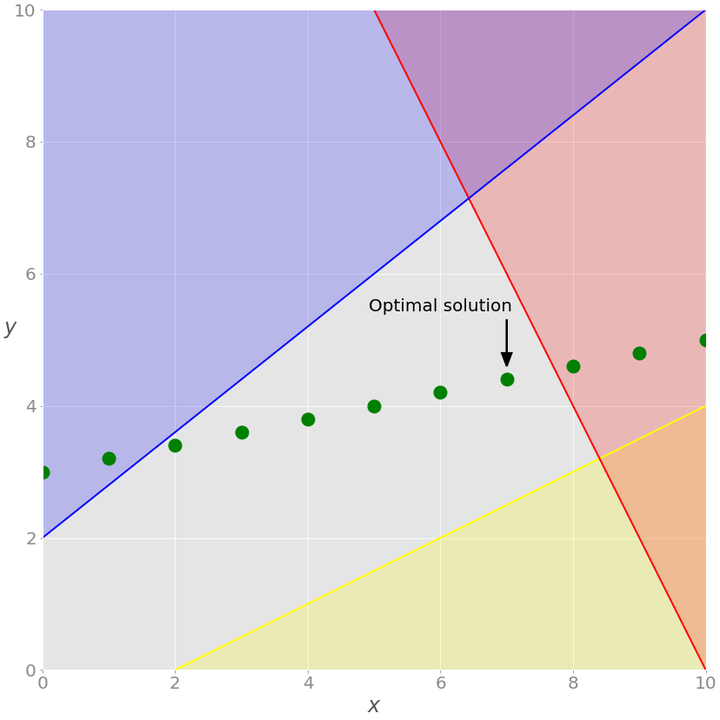GLPK 也能够解决此类问题。

### 示例 2# Define the modelmodel = LpProblem(name="resource-allocation", sense=LpMaximize)# Define the decision variablesx = {i: LpVariable(name=f"x{i}", lowBound=0) for i in range(1, 5)}# Add constraintsmodel += (lpSum(x.values()) <= 50, "manpower")model += (3 * x + 2 * x + x <= 100, "material_a")model += (x + 2 * x + 3 * x <= 90, "material_b")# Set the objectivemodel += 20 * x + 12 * x + 40 * x + 25 * x# Solve the optimization problemstatus = model.solve()# Get the resultsprint(f"status: {model.status}, {LpStatus[model.status]}")print(f"objective: {model.objective.value()}")for var in x.values():    print(f"{var.name}: {var.value()}")for name, constraint in model.constraints.items():    print(f"{name}: {constraint.value()}")

status: 1, Optimalobjective: 1900.0x1: 5.0x2: 0.0x3: 45.0x4: 0.0manpower: 0.0material_a: -40.0material_b: 0.0

 1model = LpProblem(name="resource-allocation", sense=LpMaximize) 2 3# Define the decision variables 4x = {i: LpVariable(name=f"x{i}", lowBound=0) for i in range(1, 5)} 5y = {i: LpVariable(name=f"y{i}", cat="Binary") for i in (1, 3)} 6 7# Add constraints 8model += (lpSum(x.values()) <= 50, "manpower") 9model += (3 * x + 2 * x + x <= 100, "material_a")10model += (x + 2 * x + 3 * x <= 90, "material_b")1112M = 10013model += (x <= y * M, "x1_constraint")14model += (x <= y * M, "x3_constraint")15model += (y + y <= 1, "y_constraint")1617# Set objective18model += 20 * x + 12 * x + 40 * x + 25 * x1920# Solve the optimization problem21status = model.solve()2223print(f"status: {model.status}, {LpStatus[model.status]}")24print(f"objective: {model.objective.value()}")2526for var in model.variables():27    print(f"{var.name}: {var.value()}")2829for name, constraint in model.constraints.items():30    print(f"{name}: {constraint.value()}")

• 第 5 行定义了二元决策变量 y并 y保存在字典中 y。

• 第 12 行定义了一个任意大的数 M。100 在这种情况下，该值足够大，因为您 100 每天的数量不能超过单位。

• 第 13 行说如果 y为零，则 x必须为零，否则它可以是任何非负数。

• 第 14 行说如果 y为零，则 x必须为零，否则它可以是任何非负数。

• 第 15 行说要么 yory为零（或两者都是），所以要么 xor 也 x必须为零。

status: 1, Optimalobjective: 1800.0x1: 0.0x2: 0.0x3: 45.0x4: 0.0y1: 0.0y3: 1.0manpower: -5.0material_a: -55.0material_b: 0.0x1_constraint: 0.0x3_constraint: -55.0y_constraint: 0.0

## 线性规划求解器

• GLPK

• LP Solve

• CLP

• CBC

• CVXOPT

• SciPy

• SCIP with PySCIPOpt

• Gurobi Optimizer

• CPLEX

• XPRESS

• MOSEK

## 结论## 评论